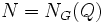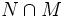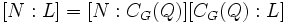# Frobenius' normal p-complement theorem

This article gives the statement, and possibly proof, of a normal p-complement theorem: necessary and/or sufficient conditions for the existence of a Normal p-complement (?). In other words, it gives necessary and/or sufficient conditions for a given finite group to be a P-nilpotent group (?) for some prime number$p$.
View other normal p-complement theorems

## Statement

Let$G$ be a finite group and$p$ be a prime number. Then, the following are equivalent:

1.$G$ has a$p$-Sylow subgroup$P$ that is conjugacy-closed in$G$: any two elements of$P$ that are conjugate in$G$ are conjugate in$P$.
2. There is a normal p-complement in$G$: a normal subgroup whose index is a power of$p$ and whose order is relatively prime to$p$. In other words, any$p$-Sylow subgroup is a retract of$G$.
3. For every non-identity$p$-subgroup$Q$ of$G$, the subgroup$N_G(Q)$ has a normal p-complement
4. For every non-identity$p$-subgroup$Q$, the quotient$N_G(Q)/C_G(Q)$ is a$p$-group.

## Proof

### (2) implies (3)

(Note: This implication uses nothing about the special nature of normalizers of$p$-subgroups, and works for all subgroups).

Given: A finite group$G$, a prime$p$, a$p$-Sylow subgroup$P$ of$G$. A normal subgroup$M$ of$G$ such that$MP = G$ and$M \cap P$ is trivial.

To prove: If$Q$ is a non-identity$p$-subgroup of$G$, then$N_G(Q)$ has a normal$p$-complement.

Proof: Let$N = N_G(Q)$. By the second isomorphism theorem, we have:$NM/M \cong N/(N \cap M)$.

Since$[NM:M]$ divides$[G:M]$, and$[G:M]$ equals the order of$P$,$[NM:M]$ is a power of$p$. Thus, so is the order of the right side,$[N:N \cap M]$.

Thus,$N \cap M$ is a normal subgroup of$N$ whose order is relatively prime to$p$ (since it is also a subgroup of$M$) and whose index is a power of$p$. Thus, it is a normal$p$-complement in$N$.

### (3) implies (4)

Given: A finite group$G$, a prime$p$, a$p$-Sylow subgroup$P$ of$G$. For any non-identity$p$-subgroup$Q$,$N_G(Q)$ has a normal$p$-complement.

To prove: If$Q$ is a non-identity$p$-subgroup of$G$, then$N_G(Q)/C_G(Q)$ is a$p$-group.

Proof: Proof: Let$N = N_G(Q)$. Let$L$ be a normal$p$-complement in$N$.

Now,$Q$ and$L$ are both normal subgroups of$N$ and since the order of$L$ is relatively prime to$p$,$Q \cap L$ is trivial. Thus,$L \le C_G(Q)$. Thus, by fact (1):$[N:L] = [N:C_G(Q)][C_G(Q):L]$.

Since the left side is a power of$p$, so are both terms of the right side. In particular,$N_G(Q)/C_G(Q)$ is a$p$-group.

### (2) implies (1)

This follows from fact (3).

### (1) implies (2)

This follows from fact (4).